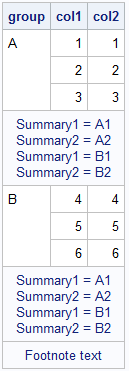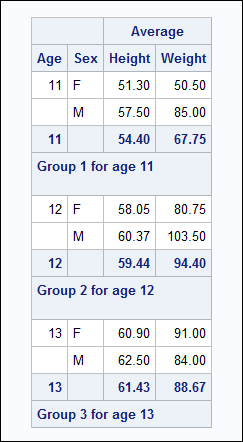## Insert lines after Group in PROC REPORT

Hello SAS experts,

I am trying to create a report that prints additional lines with extra information after each group value. The extra information is currently stored as macro variables. I can set this layout up, however, I am having a hard time telling SAS what pieces of information to put when. In the sample report below, I want the first break to only read "Summary1 = A1" and "Summary2 = A2" and the second break to read "Summary1 = B1" and "Summary2 = B2".Here is sample code with two options I've tried, neither are working how I envision they should:

`%let VALA1 = A1;%let VALA2 = A2;%let VALB1 = B1;%let VALB2 = B2;data test;input group \$ col1 col2;datalines;A 1 1A 2 2A 3 3B 4 4B 5 5B 6 6;run;proc report data=test spanrows nowd out=outrpt; columns Group col1 col2; define group / group; define col1 / display; define col2 /display; compute after group; if Group = "A" then do; line "Summary1 = &VALA1"; line "Summary2 = &VALA2"; end; if Group = "B" then do; line "Summary1 = &VALB1"; line "Summary2 = &VALB2"; end; endcomp; compute after; line "Footnote text"; endcomp;run;%Macro Test;proc report data=test spanrows nowd; columns Group col1 col2; define group / group; define col1 / display; define col2 /display; compute after group; %if Group = "A" %then %do; line "Summary1 = &VALA1"; line "Summary2 = &VALA2"; %end; %if Group = "B" %then %do; line "Summary1 = &VALB1"; line "Summary2 = &VALB2"; %end; endcomp; compute after; line "Footnote text"; endcomp;run;%Mend Test;%Test;`

The first PROC REPORT prints both lines after each group value as if it's ignoring the IF/THEN blocks. The second PROC REPORT doesn't print any lines. Does anyone have ideas for solutions? Ideally I would keep the extra information stored as macro variables, but if it works to add in as separate columns, I can do that as well.

Thank you,

Brian

1 ACCEPTED SOLUTION

Accepted Solutions

## Re: Insert lines after Group in PROC REPORT

Hi:

The issue that the OP encountered was due to the fact that with PROC REPORT, the LINE statement output is ALWAYS written. You cannot execute a LINE statement conditionally. In many ways, the LINE statement has behavior in common with the PUT statement. But, where a PUT can be executed based on a condition, the LINE statement cannot be executed based on a condition. The LINE statement is ALWAYS written and is ALWAYS written after all the other statements in the COMPUTE block have executed.

You CAN however, make a temporary variable and control the text that you write using conditions, like this:

if xx=1 then tmpvar='This is for 1';

else if xx=2 then tmpvar='This is for 2';

line tmpvar \$100.;

Or you can make separate columns and add extra breaking variables, as shown on page 28 of this paper https://www.sas.com/content/dam/SAS/support/en/technical-papers/SAS0431-2017.pdf see Output 23 for the example.

I assume you want something like this:Here's some sample code that created the above screen shot.

``````
proc sort data=sashelp.class out=class;
where age le 13;
by age;
run;

%let grp1=Group 1;
%let grp2=Group 2;
%let grp3=Group 3;

proc report data=class
column age sex ('Average' height weight);
define age / group;
define sex / group;
define height / mean f=6.2;
define weight / mean f=6.2;
break after age / summarize;
compute after age;
length want_this1 \$100 want_this2 \$1;
want_this2=' ';
if age = 11 then do;
want_this1=catx(' ',"&grp1","for age",age);
lg2=1;
end;
else if age = 12 then do;
want_this1=catx(' ',"&grp2","for age",age);
lg2=1;
end;
else if age = 13 then do;
want_this1=catx(' ',"&grp3","for age",age);
lg2=0;
end;
line want_this1 \$100.;
line want_this2 \$varying. lg2;
endcomp;
run;
``````

In the above example, want_this1 and want_this2 are the temporary variables. The string to print is assigned conditionally to want_this1. Then, want_this2 is just a blank. Notice how there is a blank line under Group 1 and Group 2, but not under Group 3. Want_this2 was assigned a value of blank, but the printing of the line was controlled by using a length of either 1 or 0. For the LINE statement, if you use \$VARYING. format with a length of 0, then PROC REPORT suppresses writing the LINE.

cynthia

3 REPLIES 3

## Re: Insert lines after Group in PROC REPORT

The second bit using macro code doesn't show anything because you are using macro %if with data set values. The macro facility generates code and all the % statement elements are compiled and resolved before the procedure starts to execute. The macro facility does not look at the values of the data set variables only of macro variables.

This seems to do what I think you are asking for:

```proc format library=work;
value \$val1_
'A'="Summary1 = &VALA1"
'B'="Summary1 = &VALB1"
;
value \$val2_
'A'="Summary2 = &VALA2"
'B'="Summary2 = &VALB2"
;
run;

proc report data=test spanrows nowd out=outrpt;
columns Group col1 col2;
define group / group;
define col1 / display;
define col2 /display;
compute after group;
line Group \$val1_.;
line Group \$val2_.;
endcomp;
compute after;
line "Footnote text";
endcomp;
run;```

Note that you cannot define custom formats that end in a digit, hence the _ I used.

## Re: Insert lines after Group in PROC REPORT

Hi:

The issue that the OP encountered was due to the fact that with PROC REPORT, the LINE statement output is ALWAYS written. You cannot execute a LINE statement conditionally. In many ways, the LINE statement has behavior in common with the PUT statement. But, where a PUT can be executed based on a condition, the LINE statement cannot be executed based on a condition. The LINE statement is ALWAYS written and is ALWAYS written after all the other statements in the COMPUTE block have executed.

You CAN however, make a temporary variable and control the text that you write using conditions, like this:

if xx=1 then tmpvar='This is for 1';

else if xx=2 then tmpvar='This is for 2';

line tmpvar \$100.;

Or you can make separate columns and add extra breaking variables, as shown on page 28 of this paper https://www.sas.com/content/dam/SAS/support/en/technical-papers/SAS0431-2017.pdf see Output 23 for the example.

I assume you want something like this:Here's some sample code that created the above screen shot.

``````
proc sort data=sashelp.class out=class;
where age le 13;
by age;
run;

%let grp1=Group 1;
%let grp2=Group 2;
%let grp3=Group 3;

proc report data=class
column age sex ('Average' height weight);
define age / group;
define sex / group;
define height / mean f=6.2;
define weight / mean f=6.2;
break after age / summarize;
compute after age;
length want_this1 \$100 want_this2 \$1;
want_this2=' ';
if age = 11 then do;
want_this1=catx(' ',"&grp1","for age",age);
lg2=1;
end;
else if age = 12 then do;
want_this1=catx(' ',"&grp2","for age",age);
lg2=1;
end;
else if age = 13 then do;
want_this1=catx(' ',"&grp3","for age",age);
lg2=0;
end;
line want_this1 \$100.;
line want_this2 \$varying. lg2;
endcomp;
run;
``````

In the above example, want_this1 and want_this2 are the temporary variables. The string to print is assigned conditionally to want_this1. Then, want_this2 is just a blank. Notice how there is a blank line under Group 1 and Group 2, but not under Group 3. Want_this2 was assigned a value of blank, but the printing of the line was controlled by using a length of either 1 or 0. For the LINE statement, if you use \$VARYING. format with a length of 0, then PROC REPORT suppresses writing the LINE.

cynthia

## Re: Insert lines after Group in PROC REPORT

Cynthia, thank you for explaining the causes of why I was getting "unexpected" behavior and posting a solution! This is exactly what I was looking for and works when I extend the logic to my production code.

-Brian

Discussion stats
• 3 replies
• 9114 views
• 1 like
• 3 in conversation